# REPT Function In ExcelArticle byExcelMojo Team## What Is REPT Function In Excel?

The REPT function in Excel helps users repeat a text string, characters, symbols, etc., a required number of times. The function is used during building histograms during financial modeling by converting data points directly into the required number of repeated characters.

The REPT Excel Function is an inbuilt text function, so we can insert the formula from the “Function Library” or enter it directly in the worksheet. For example, the below table contains a list of text values and the number of times we require to repeat them.

To use the REPT Function In Excel, select cell C2, enter the formula =REPT(A2,B2), press “Enter”, and drag the formula from cell C2 to C5 using the fill handle.

The output is shown above. The column C formulas repeat the given texts the stipulated number of times, whether they are special characters, texts, or numeric values. Column D is for our reference.

###### Key Takeaways
• The REPT function in Excel repeats the provided text the stipulated number of times.
• Users can utilize the REPT() to fill cells with values of required lengths. And the function enables them to create in-cell histograms, representing the given data with repeated characters, such as solid squares.
• Excel will not evaluate the function if a special character, entered as the text argument, is not in double-quotes. On the other hand, numbers entered as the text argument does not require double-quotes.
• We can use REPT() with other Excel functions, such as SUM, CHAR, UNICHAR, VLOOKUP, SUBSTITUTE, and LEN.

### REPT() Excel Formula

The syntax of the REPT Excel formula is:

The arguments of the REPT Excel formula are:

• text: The text, character, or symbol to repeat. It is a mandatory argument.
• number_times: A positive number specifying the number of times to repeat the provided text. It is a mandatory argument.

### How To Use REPT Excel Function?

We can use the REPT function in Excel using 2 methods, namely,

1. Access from the Excel ribbon.
2. Enter in the worksheet manually.

#### Method #1 – Access from the Excel ribbon

First, choose an empty cell → select the “Formulas” tab → go to the “Function Library” group → click the “Text” option drop-down → select the “REPT” function, as shown below.

The “Function Arguments” window appears. Enter the argument values in the “Text”, and “Number_times” fields → click “OK”, as shown below.

#### Method #2 – Enter in the worksheet manually

1. Select an empty cell for the output.
2. Type =REPT( in the cell. [Alternatively, type =RE and select the REPT function from the Excel suggestions.]
3. Enter the arguments as cell values or cell references in Excel.
4. Close the brackets and press Enter to view the repeated text.

Let us take an example to understand this function.

We will repeat a list of texts using REPT function in Excel.

The below table shows a list of texts and the number of times they should repeat.

The steps to repeat the text values using REPT function in Excel are,

1. First, select cell C2, enter the formula =REPT(A2,B2), and press Enter.

[The REPT() accepts the phrase “Hello World!” as the argument text and the value 3 as the second argument, number_times. And it returns the phrase “Hello World!” thrice in the target cell C2.]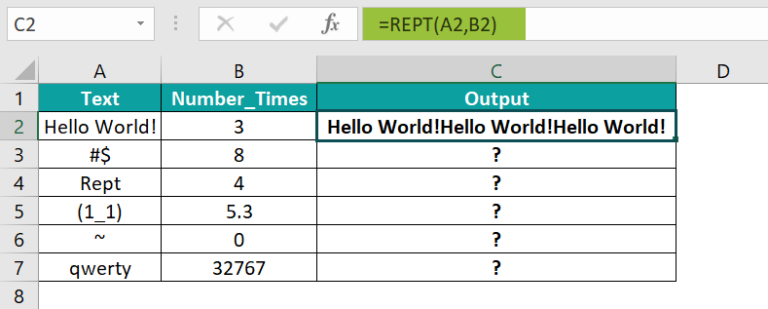[Alternatively, choose cell C2 and apply the REPT() using the option in the FormulasTextREPT, which will open the Function Arguments window.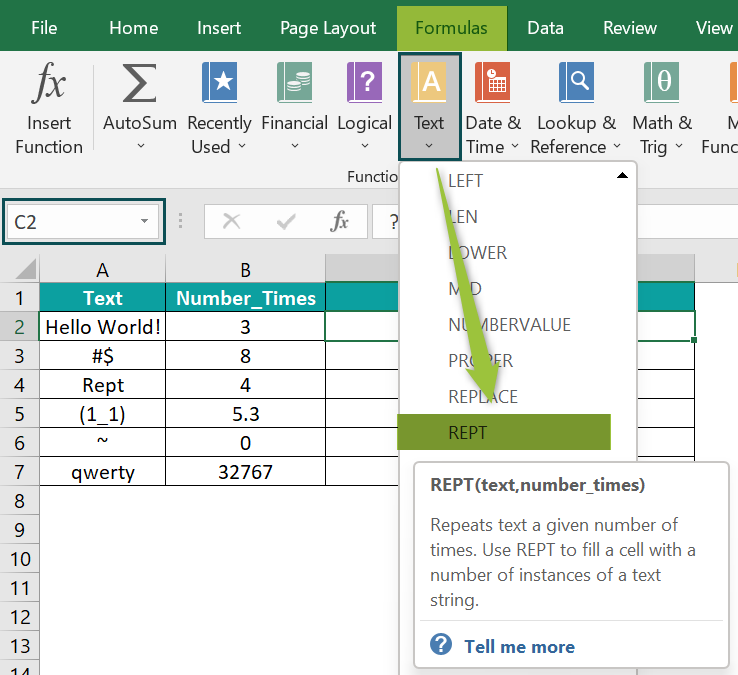Next, enter the values of the two arguments, Text and Number_times, in the Function Arguments window.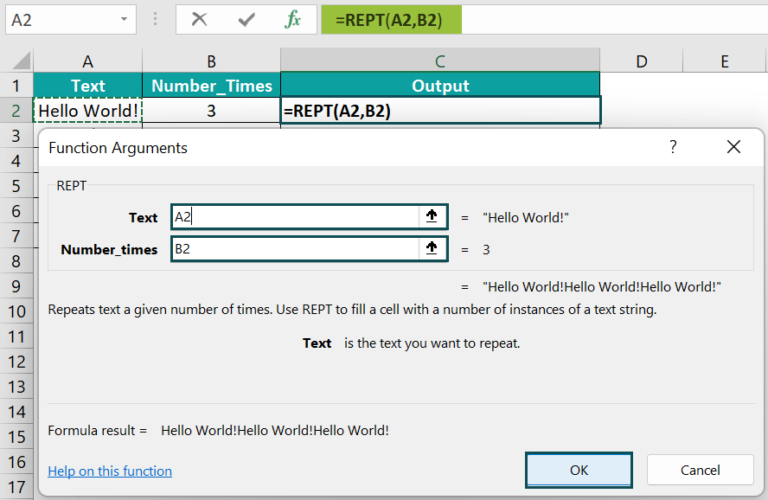And once we click OK, we can view the repeated text as the output in the target cell C2.]

2. Select cell C3, enter the formula =REPT(“#\$”,8), and press Enter.

[The above REPT() accepts the argument values directly and returns the output with the special symbols repeated eight times.]

[Note: If we provide the special symbols as the text argument without the double quotes, Excel will show a warning message indicating some issue with the formula.]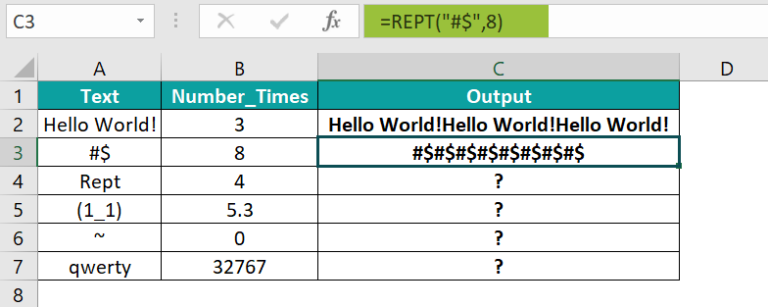3. Now, select cell C4, enter the formula =REPT(A4,4), and press Enter.

[Here, the REPT() returns the text Rept repeated four times as the output in cell C4.]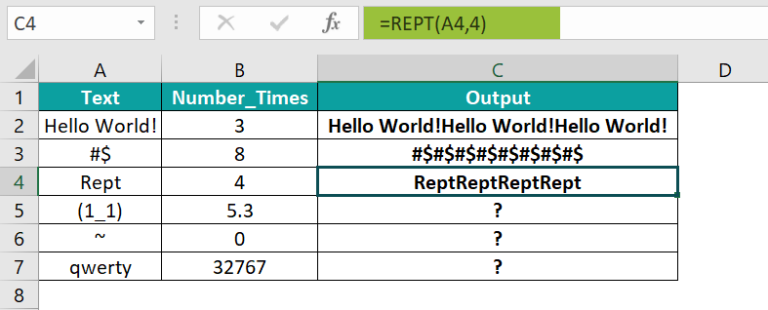4. Select cell C5, enter the formula =REPT(“(1_1)”,B5), and press Enter.

[The second argument in the above REPT() is a decimal number, 5.3. So, the function truncates it and considers the argument value as 5, thus repeating the given text five times.]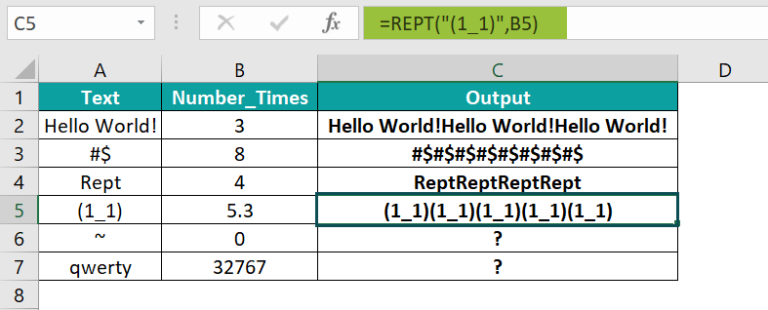5. Next, select cell C6, enter the formula =REPT(A6,B6), and press Enter.

[The second argument in the above REPT() is 0, and thus the function returns an empty text as the output in the target cell C6.]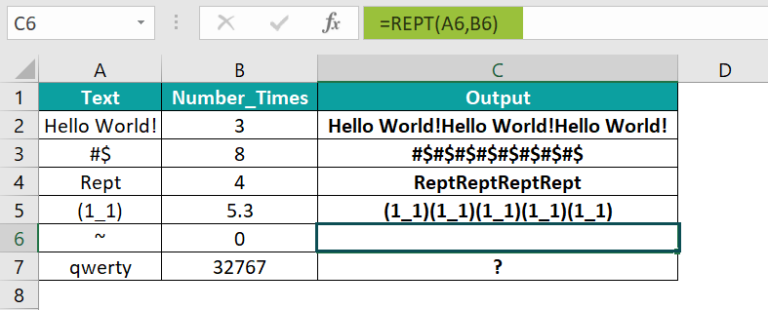6. Select cell C7, enter the formula =REPT(A7,B7), and press Enter.

[The second argument in the above REPT() is 32767. And if the text “qwerty” repeats 32767 times, it will exceed the character limit for the REPT(), thus resulting in an error.]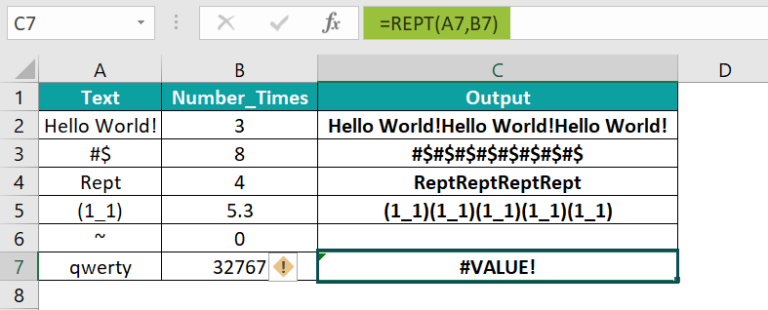The output is shown above.

### Examples

We will understand advanced scenarios using the REPT function in Excel examples.

#### Example #1

We will display the locker number data as confidential and replace the numbers in the string with *’, leaving the text “LB” visible, using the REPT, SUM, LEN, and SUBSTITUTE excel function.

The below table contains a list of bank customers and their locker numbers in columns A and B.

The steps to keep the locker number confidential, and replace it with repeated “*” using the REPT, LEN, SUBSTITUTE and SUM() Excel function are,

• Step 1 : Select cell C2, enter the formula =”LB”&REPT(“*”,SUM(LEN(B2)LEN(SUBSTITUTE(B2,{1,2,3,4,5,6,7,8,9,0},)))), and press Enter.
• Step 2: Drag the formula from cell C2 to C7 using the fill handle.

We get the output as shown above.

[Output Observation: Let us consider the target cell C7 formula to see how it works.

The first LEN Excel function returns the length of cell B7 value, LB44219836, 10. Then, the SUBSTITUTE() substitutes every occurrence of each digit (from the array) in the cell B7 value with “”. And it returns the output {“LB4429836”,”LB4419836”,”LB4421986”,”LB219836”,”LB44219836”,”LB4421983”, ”LB44219836”,”LB4421936”,”LB4421836”,”LB44219836”}. The LEN() then returns the length of each string in the above array, {9,9,9,8,10,9,10,9,9,10}. Then the expression inside the SUM() finds the difference between the length of cell B7 value, 10, and each value in the above array, returning {1,1,2,0,1,0,1,1,0}. The SUM() adds the array values and gives the value 8 as the output.

The REPT() takes ‘*’ as the text argument and the value 8 as the number_times argument to repeat the symbol eight times. And finally, the formula adds the phrase “LB” before the REPT() output.

Thus, the above formula replaces the number of digits in the given string with an equal number of asterisks.]

#### Example #2

We will use the REPT function in Excel with UNICHAR() and VLOOKUP().

The first table in the image below shows a brand’s monthly star ratings. We will show the star ratings in the second table for a specific quarter in the form of stars based on the first table data.

The steps to repeat * using the REPT function in Excel with UNICHAR() and VLOOKUP() are,

• Step 1: Select cell F2, enter the formula =REPT(UNICHAR(9733),VLOOKUP(E2,A2:B13,2,0)), and press Enter.
• Step 2: Drag the formula from cell C2 to C4 using the fill handle.

We get the output as shown above.

[Output Observation: We shall consider the target cell C4 formula to understand how it works. First, the UNICHAR() returns the star symbol. Then, the VLOOKUP() looks up the star rating for June in the first table to return the valueof 4.6. However, as 4.6 is not an integer, the REPT() truncates the value to 4. And thus, the function returns four stars as the output.]

#### Example #3

We will display the sales values in the form of in-cell histograms using the REPT and CHAR function in Excel.

The following table contains a list of items and their sales figures in columns A and B.

The steps to display in-cell histogram using the REPT() and CHAR() are,

• Step 1: Select cell C2, enter the formula =REPT(CHAR(110),B2/500), and press Enter.
• Step 2: Drag the formula from cell C2 to C6 using the fill handle.
• Step 3: Finally, select cells C2:C6 → click HomeFontWingdings.

We will get the following output, i.e., it will display the character n as a solid square.

[Output Observation: Let us consider the target cell C6 formula to see how it works.

First, the CHAR() returns the character n. Now, the logic is to show the sales values in units of 500, and thus we divide column B values by 500. So, the expression B6/500 in cell C6 evaluates as 4.62. However, as the REPT() takes only an integer as the second argument, the value 4.62 gets truncated to 4. Thus, the REPT() displays the character n four times as the output. And finally, applying the font Wingdings displays the character n as a solid square.]

### Important Things to Note

• If the REPT() second argument is a decimal number, then the function will consider only the whole number or the integer.
• When we provide the second argument as 0, the REPT function returns an empty text or a blank cell.
• The maximum number of characters we can enter in an Excel cell is 32,767. If the REPT() results in a value containing more than 32,767 characters, we will get a #VALUE! error.
• When the number_times argument is a negative number, we get a #VALUE! error.

1. Is there a repeat function in Excel?

We have a repeat function in Excel called the REPT(), which is available in the Formulas tab. We need to click Formulas Text REPT to use it.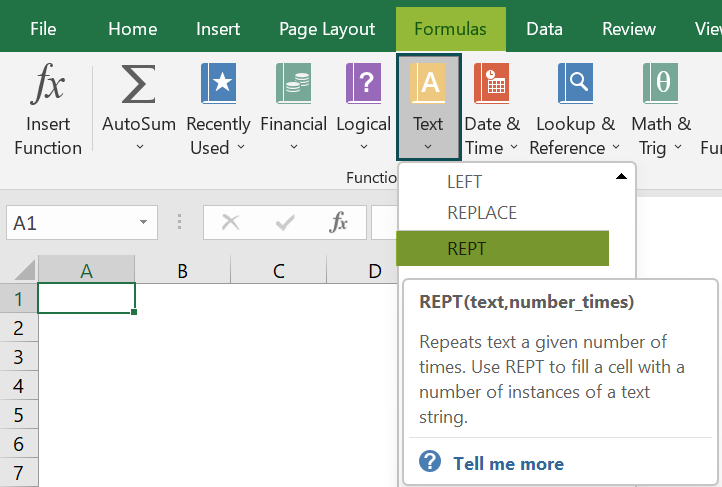2. How can we build a tally chart with REPT() in Excel?

We can build a tally chart in units of 5, with the REPT function in Excel using the below formula:
=REPT(“tttt”,QUOTIENT(…))&REPT(“I”,MOD(…))

For example, the below table contains a list of inventory items and their quantity details.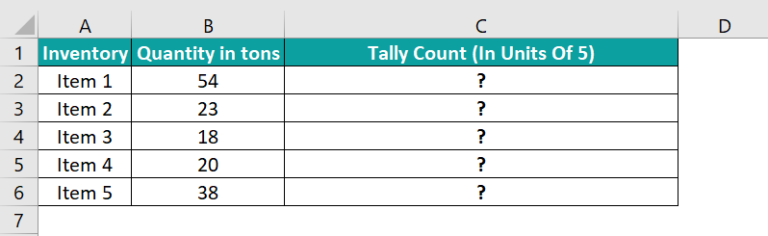The steps to create a tally chart using the REPT(), QUOTIENT(), and MOD() functions are:

• 1: Select cell C2, enter the formula =REPT(“tttt”,QUOTIENT(B2,5))&REPT(“I”,MOD(B2,5)), and press Enter.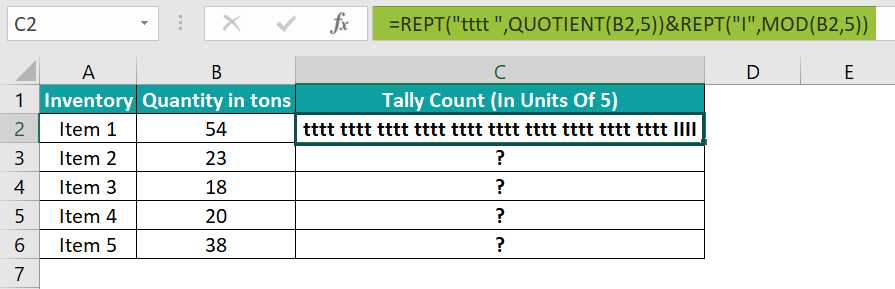• 2: Drag the formula from cell C2 to C6 using the fill handle.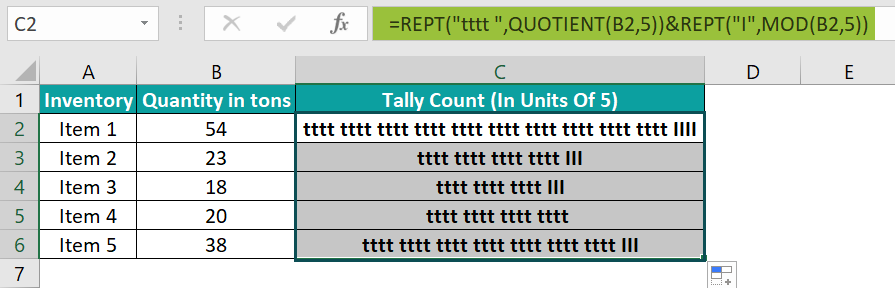[Let us look at the target cell C6 formula to understand how the formula works.
First, the QUOTIENT() output is the quotient of the expression 38/5, 7. So, the first REPT() returns the text “tttt” seven times. Then the MOD() returns the remainder of the expression 38/7, 3. And so, the second REPT() output is the text “I” repeated thrice. Thus, the formula shows the quantity value 38 as 28 t’s in units of 5 and three I’s.]

• 3: Finally, to create a tally chart from the formula results, select cells C2:C6. And then click HomeFontCentury Gothic, as shown below.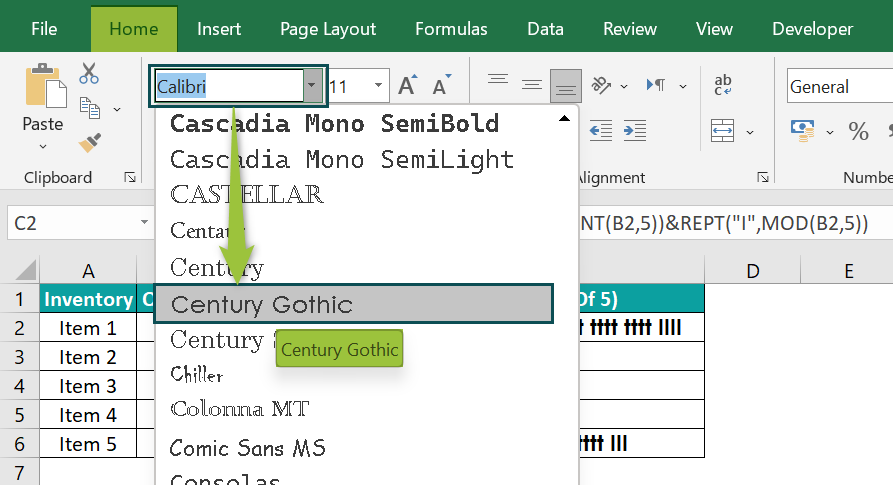We get the output as shown below, i.e., it shows a single complete tally sign, denoted by the count of five instances.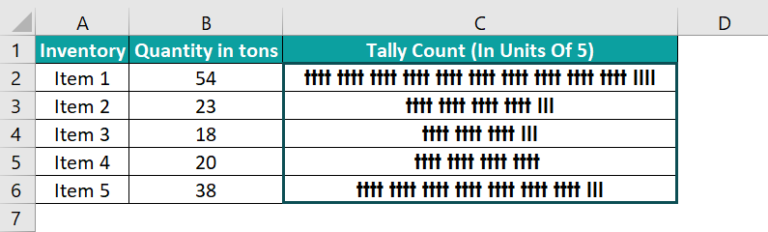3. Why is my REPT function in Excel not working?

The REPT function in Excel may not work due to the following reasons:
• If the directly supplied text or special characters as the first argument, text, to the REPT() are not in double quotations.
The second argument we supplied to the REPT() is 0, or a negative number.
And if the REPT() evaluates to a value containing more than 32,767 characters.